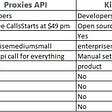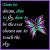# The intersection of two arrays II solution ~ Leetcode

Today, we will take a look at how we can solve an intersection of two arrays problem in Leetcode. I am not a great programmer but I can give you an average solution for the question. I have provided the question and answer below,

Given two arrays, write a function to compute their intersection.

Example 1:

`Input: nums1 = [1,2,2,1], nums2 = [2,2]Output: [2,2]`

Example 2:

`Input: nums1 = [4,9,5], nums2 = [9,4,9,8,4]Output: [4,9]`

Note:

• Each element in the result should appear as many times as it shows in both arrays.
• The result can be in any order.

In the question, we have to note the key points given under “Note”. The elements in the output should appear as many times as it appeared after the intersection of two arrays.

I will break down the algorithm for your understanding,

• Create two counters representing the number of times each element appeared in the array for both arrays.
• Iterate through the keys of the first counter and check if the key exists in the second counter.
• If the key exists, add the key to the output array. It has to be extended with the minimum number of times the key appeared in either of those arrays.

Below is the code of the program,

`from collections import Counterclass Solution(object):    def intersect(self, nums1, nums2):        """        :type nums1: List[int]        :type nums2: List[int]        :rtype: List[int]        """        counter_1 = Counter(nums1) #creates a dictionary of key value pairs        counter_2 = Counter(nums2)        output = [] #output array to store the ouput        for key in counter_1.keys():            if key in counter_2.keys():                output.extend([key]*min(counter_1[key], counter_2[key]))                                                                                      return output`

# Improvements

You can improve the algorithm in case your inputs are different from the one which is given in the above example. In case you have given two arrays where one is much smaller in size, you can use that array in the for loop so that the number of iterations can be reduced.

Example 3:

`Input: nums1 = [1,1], nums2 = [2,2,5,6,9,0,5,3,4]Output: []`

In the above example, you can see that there is no point in iterating through the second array as the number in nums1 does not exist in nums2. If you iterate through nums1, you have to iterate only once. However, if you iterate through nums2, you have to iterate 9 times. I hope you got the point.

Happy Learning!

--

--

--

## More from Abinaya Rajesh

Love podcasts or audiobooks? Learn on the go with our new app.

## Building a minimum viable product (MVP)## Here’s why I converted my blog site to become a PWA (Progressive Web App)## Traefik Ingress with Kubernetes on AWS## Testing Terraform code with Go and Terratest## Writing Clean Code, Part I — The art of naming stuff## Kimura Framework Vs. Proxies API## 6 go-to questions you can ask at the end of a behavioral interview — and stand out in the process## Meta New Grad Recruiting Reflection